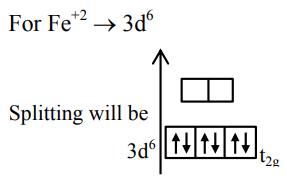# Spin only magnetic moment of an octahedral complexQuestion:

Spin only magnetic moment of an octahedral complex of $\mathrm{Fe}^{2+}$ in the presence of a strong field ligand in BM is :

1. 4.89

2. 2.82

3. 0

4. 3.46

Correct Option: , 3

Solution:

In presence of SFL $\Delta_{0}>\mathrm{P}$ means pairing occurs therefore$\therefore$ No of unpaired $\mathrm{e}^{-}(\mathrm{s})=0$

$\therefore \mu=\sqrt{n(n+2)} B M=0$

$\left[n=\right.$ No of unpaired $\left.e^{-}(s)\right]$

In $\mathrm{NiCl}_{2} \mathrm{Ni}^{+2}$ is having configuration $3 \mathrm{~d}^{8}$

$\therefore \quad$ Number of unpaired electron $=2$

After formation of oxidised product

$\left[\mathrm{Ni}(\mathrm{CN})_{6}\right]^{-2} \mathrm{Ni}^{+4}$ is obtained

$\mathrm{Ni}^{+4} \Rightarrow 3 \mathrm{~d}^{6}$ and $\mathrm{CN}^{-}$is strong field ligand

$\therefore$ number of unpaired electrons $=0$

$\therefore$ The charge is $2-0=2$